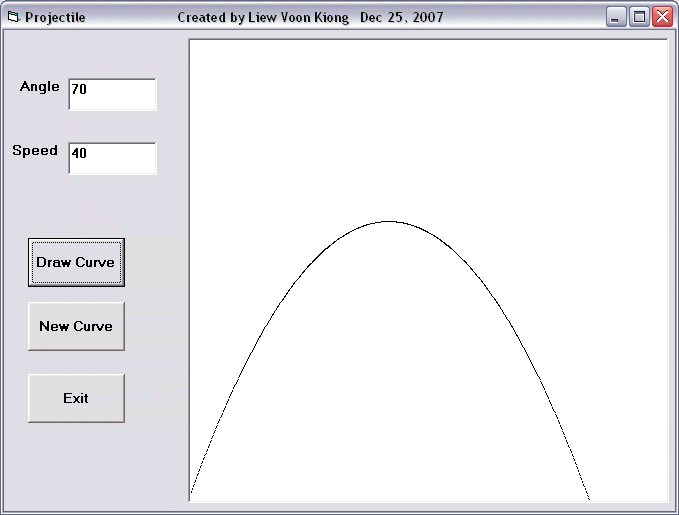# Projectile Simulation Program

This is a Visual Basic program that can plot the path of a projectile, the basic concept of missile launching. This path is determined by the launching angle and speed. The formula is y=(Vsin a) t- 1/2(gt2) and x=(Vcosa)t, where V=launching speed and a is the launching angle, g is acceleration due to gravity(9.8n ms-2) while t is time.

In this program, we use a picture box for drawing the curve . The command Pset is used to plot the curve. Pset is a method that draw a point on the screen. Using loop such as the do loop will connect all the points into a line.

### The Interface### The Code

Private Sub cmd_Draw_Click()

Dim x, y, v, t, a As Single
v = Txt_Speed.Text
a = Txt_Angle.Text
Pic_Curve.Cls

Do
t = t + 0.01
y = v * Sin(a * 3.141592654 / 180) * t - 4.9 * (t ^ 2)
x = v * Cos(a * 3.141592654 / 180) * t
Pic_Curve.PSet (x, 120 - y)
If x > 120 Then
Exit Do
End If

Loop
End Sub